# MCQs on Electric Circuits

##### Page 42 of 63. Go to page 1 2 3 4 5 6 7 8 9 10 11 12 13 14 15 16 17 18 19 20 21 22 23 24 25 26 27 28 29 30 31 32 33 34 35 36 37 38 39 40 41 42 43 44 45 46 47 48 49 50 51 52 53 54 55 56 57 58 59 60 61 62 63
01․ In given figure, the value of resistance R in Ω is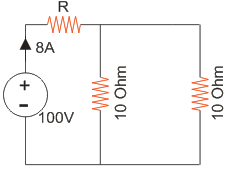2.5 Ω.
5 Ω.
7.5 Ω.
10 Ω.

The given circuit can be simplified by replacing voltage source by equivalent current source as below,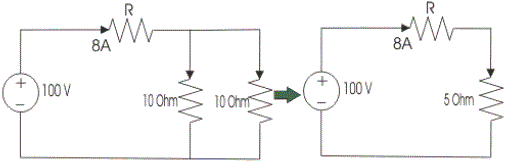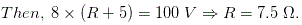02․ A 35 V source is connected to a series circuit of 600 Ω and R as shown. If a voltmeter of internal resistance 1.2 kΩ is connected across 600 Ω resistor, it reads 5 V. The value of R is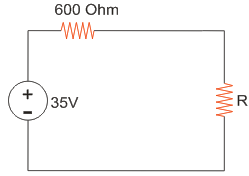2.4 k Ω.
1.2 k Ω.
3.6 k Ω.
7.2 k Ω.

As the voltmeter of internal resistance 1.2 k Ω is connected across the 600 Ω resistor and it gives 5 V reading.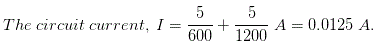As supply voltage is 35 V, the voltage across resistor R is 35 - 5 = 30 V.

03․ A certain network consists of large number of ideal linear resistances, one of which is designated as R and two constant ideal source. The power consumed by R is P1 when only the first source is active and P2 when only second source is active. In both sources are active simultaneously then the power consumed by R is
P1 ± P2 .
(√P1 ± √P2)2.
√P1 ± √P2.
(P1 ± P2 )2.

When first source is active, power P1 = i12R; where i1 is the current flowing when only the first source is active. When the second source is active, power P2 = i22R; where i2 is the current flowing when only the second source is active. When both sources are active current flowing through R is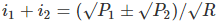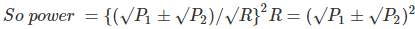04․ In the circuit given, I = 1 A for Is = 0. What is the value of I for Is = 2 A ?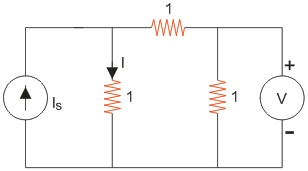4 A.
3 A.
2 A.
1 A.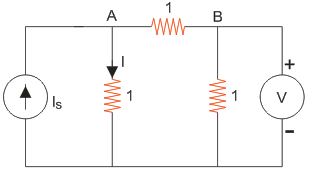When Is = 0, VB = 2V Applying KCL at node A, VA = 2V Therefore, I = Is = 2A.

05․ In the circuit shown below, what is the voltage across 5 Ω resistor?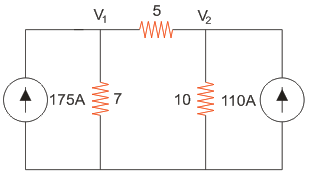-28.4 V.
34.4 V.
-27.4 V.
None of themApplying KCL at the nodes, V1 = -1185.22 V and V2 = -1156.82 V Therefore the voltage across 5Ohm resistor is -28.4V.

06․ For the circuit shown in the given figure the current I is given by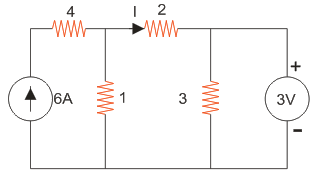2 A.
1 A.
3 A.
4 A.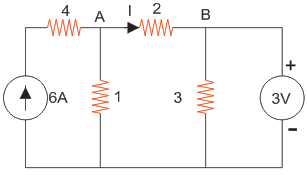VB = 3V Applying KCL at node A, VA = 5V Therefore, I = (5-3)/2 = 1A

07․ For the circuit given in the figure the power delivered by the 2 V source is given by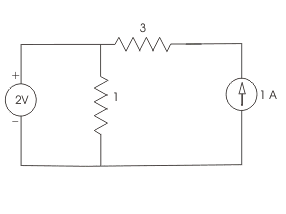4 W.
2 W.
- 2 W.
- 4 W.

Use the superposition theorem. This will give the power delivered by the voltage source without considering the current source. Consider the 2V voltage source with the current source open-circuited. Hence no current pass through the 3 Ohm resistor. So current in the circuit is 2/2 = 1A. So power delivered by the 2V source is V×I = 2×1 = 2W

08․ In the circuit shown in the figure, the value of Vs is 0, when I = 4 A. The value of I, when Vs = 16 V, is6 A.
12 A.
10 A.
8 A.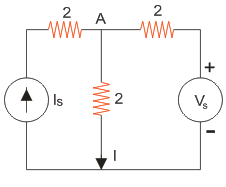If Vs = 0, then it is short circuited and I = 0.5Is. So, Is = 2×4 = 8A. When Vs = 16 V, Applying KCL at point A, VA = 16V. So, I = VA/2 = 8A.

09․ Consider the following circuit. In this circuit, when Vs = 3 V, I = 4 A, what is the value of I when Vs = 12 V?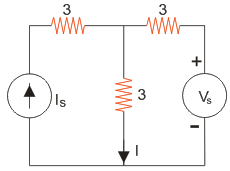7 A.
10 A.
15 A.
20 A.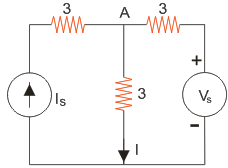When, VS = 3V, applying KCl at node A, VA = 12V and IS = 7A. When, VS = 12V, no current will flow through the right hand side branch and IS = I = 7A.

10․ In the figure given, the value of R is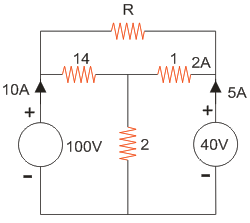12 Ω.
18 Ω.
24 Ω.
10 Ω.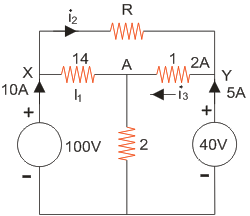Applying KCL at node A, VA = 30V; I1 = 5A; i2 = 5A; i3 = 10A; Also, potential difference between X-Y is 100 - 40 = 60V. Therefore, R = 60/5 = 12 Ohm.

<<<4041424344>>>